# Automaton, probabilistic

(diff) ← Older revision | Latest revision (diff) | Newer revision → (diff)

stochastic automaton

A generalization of a finite automaton (cf. Automaton, finite) in which the transition and the output functions are random functions. In other words, a probabilistic automaton may be defined as a system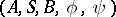where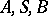are finite alphabets having the same meaning as for finite automata, while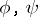are random functions mappingintoand, respectively, and are represented by systems of probability measures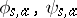, defined for allfromandfrom, onand, respectively. These measures are usually given by a stochastic matrix (cf. Automata, methods of specification of). If these probability measures can assume only two values 0 and 1, the concept of a probabilistic automaton is practically identical with that of a deterministic automaton. Autonomous probabilistic automata without output are essentially equivalent to discrete Markov chains. The functioning of a probabilistic automaton is determined similarly to that of a non-deterministic automaton, the initial state being determined by giving a probability measureon. If the probabilistic automaton is in statewith a certain probabilityand accepts the input letter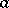, it will, with probability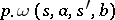pass into state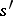, and will output the letter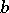of the output alphabet.

As with finite automata, probabilistic automata can be classified into transformers and acceptors, in accordance with their type of behaviour. In the former case the automaton operates by transforming input words into output words and into words in the alphabet of states with certain probabilities. If the words are of the same length, these probabilities form a probability measure, since such behaviour may be regarded as giving a countable system of such measures. In the latter case, a subset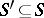of final states and a numberfrom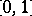, called the cut point, are given. An event which is representable by the probabilistic acceptor, whereis a random function mapping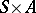intoand is defined by a system of probability measures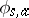defined on, consists of all words overunder the effect of which the automaton passes into one of the final states with probability at least. As distinct from finite automata, probabilistic automata can represent a continuum class of events. Moreover, even a single probabilistic automaton may represent a continuum class of events ifis varied. If the input alphabet is a one-letter alphabet, each probabilistic automaton merely represents a countable class of events, which also includes irregular events in the general case. At special cut points, the so-called isolated points, the probabilistic automaton represents only regular events. A numberfrom the segmentis known as an isolated cut point for this automaton if there exists a positive numbersuch that for any input word the probability of transforming the probabilistic automaton by this word into its final state differs by at leastfrom.

Most of the concepts and problems typical of finite automata may be generalized, in different variants, to probabilistic automata. Many of the concepts and problems preserve the properties of finite automata. For instance, it is possible to introduce the concept of equivalence of states so as to retain the well-known theorem on the possibility of differentiating between states by means of a simple experiment (cf. Automata, experiments with). On the other hand, unlike finite automata, whose minimal form is unambiguously defined (up to an isomorphism), a continuum of equivalent minimal probabilistic automata may exist for a given probabilistic automaton.

There are different ways and means of setting up probabilistic automata. For instance, a probabilistic automaton may be represented by a deterministic automaton with two inputs into one of which is fed a random sequence of input letters. Probabilistic automata are mathematical models of many real mechanisms and are used in studying the behaviour of living organisms.

How to Cite This Entry:
Automaton, probabilistic. Encyclopedia of Mathematics. URL: http://encyclopediaofmath.org/index.php?title=Automaton,_probabilistic&oldid=18171
This article was adapted from an original article by V.B. KudryavtsevYu.I. Yanov (originator), which appeared in Encyclopedia of Mathematics - ISBN 1402006098. See original article